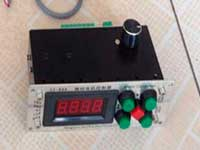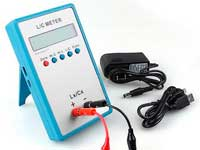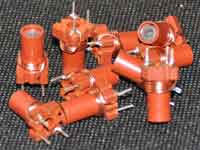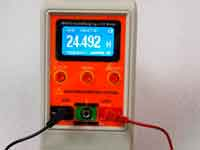Toroidal inductor calculation - 3.0 out of 5 based on 1 vote
Rating 3.00 (1 Vote)

Toroidal inductor calculationToroidal coils without a magnetic core are used very rarely. The magnetic field of a coil is almost completely centered inside of the toroid, with this kind of design has a low stray field and, accordingly, this coil is little affected by external fields even without a shield. To calculate the inductance of a toroid of circular cross-section we can take the formula for calculating the inductance of the solenoid (formula). S must be replaced by the cross-sectional area of the toroid and l is the average length of the circumference of the toroid. At the end of such manipulations, we will get the following formula:The notation is clear from the figure, all dimensions are in meters, inductance in Henry, N is the number of turns of the coil. The formula has not only of academic interest but also can be used for practical calculations of the toroidal coils with round cross section.However, in Amateur radio practice, we often deal with toroids having a square cross section, although with a large number of turns, the cross-section of a coil becomes more look like an ellipse. Experimentally it was found, that in case of the toroid with a square cross section, it is better to use empirical formulae for coil on a ferrite ring, only the permeability in this case is equal to one. It is implemented in the program Coil32.

Сomments from anonymous guests are enabled with moderation.

Coil Winding MachineUS \$187.53
The system consists of individual components including a controller, a stepping motor, a foot switch, power adapter and output shaft. This design makes it very easy for you to integrate the system into your own project.

LC Meter LC200AUS \$37.99
Measuring Capacitance Range:
0.01 pF - 100mF
Measuring Inductance Range:
0.001 uH - 100H
Measurement accuracy:1%.

RF InductorsUS \$6.43
Variable Ferrite Core Inductor coil set 3.5Turns 25-100MHZ

Auto Ranging LCR MeterUS \$63.07
Meter can measure 0.00pF to 100.00mF capacitance, 0.000uH to 100.00H inductance and 0.00? to 10.000M? resistance. Very suitable for small value capacitor and inductor measurement.